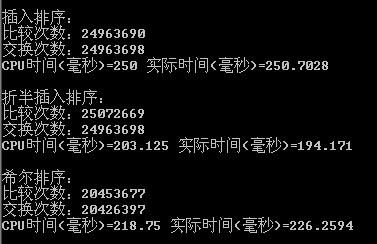# 波斯码BOSSMA Information Technology

## c#排序算法之折半插入排序

```static void BinaryInsertionSort(int[] intArr)
{
int numberOfLoop = 0;
int numberOfSwap = 0;

for (int i = 1; i < intArr.Length; i++)
{
//处理第i个数，i之前的数字排列是有序的
int j;
int temp = intArr[i];

int low = 0;
int high = i - 1;
while (low <= high)
{
numberOfLoop++;

//有序部分的的中间位置
int middle = (low + high) / 2;
if (intArr[middle] > temp)
{
high = middle - 1;
}
else
{
//最后low就是大于temp的第一个数的前一个位置
low = middle + 1;
}
}

//找到插入的位置了：low
for (j = i - 1; j >= low; j--)
{
numberOfLoop++;
numberOfSwap++;
intArr[j + 1] = intArr[j];
}

//最后一个位置设置成要排序数
numberOfSwap++;
intArr[low] = temp;
}

DisplaySortResult(numberOfLoop, numberOfSwap, intArr);

}
```【上一篇】 【下一篇】

### 发表评论

##### 相关文章